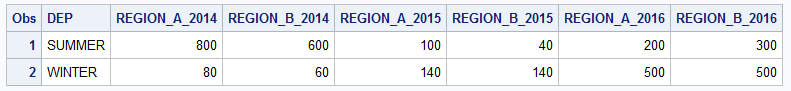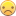## RENAMING variables with labels when labels are identical

Hello,

The macro below is very useful when you run it after a proc report because it replaces the meaningless _Ci_ with the variable labels.

However, it bugs with the following example because the numeric variables have the same label ! The Year is no longer in the output file. Would it be possible to modify the macro and add  a suffix (and get for example: REGION_A_1,  REGION_B_1, etc.) so the macro can always run;

data F1;

label x='REGION_A' y='REGION_B'; FORMAT DEP \$8.;

DEP="SUMMER" ; x=200 ; Y=300; Year=2016; output;

DEP="SUMMER"; x=100; Y=40;Year=2015; output;

DEP="SUMMER"; x=800; Y=600; Year=2014; output;

DEP="WINTER" ; x=500 ; Y=500; Year=2016; output;

DEP="WINTER"; x=140; Y=140;Year=2015; output;

DEP="WINTER"; x=80; Y=60; Year=2014; output; run;

proc report data=F1 nowd out=F2(drop=_BREAK_);

column DEP year, (X Y);

define dep / group;

define year / across; run;

%renlabel(F2) does not work

****************************************************************************

%macro renlabel(dsn);

%let dsid=%sysfunc(open(&dsn));

%let cnt=%sysfunc(attrn(&dsid,nvars));

%do i= 1 %to &cnt;

%let var&i=%sysfunc(varname(&dsid,&i));

%let lab&i=%sysfunc(varlabel(&dsid,&i));

%if &&lab&i = %then %let lab&i=&&var&i;

%end;

%let rc=%sysfunc(close(&dsid));

proc datasets;

modify &dsn;

rename

%do j = 1 %to &cnt;

%if &&var&j ne &&lab&j %then %do;

&&var&j=&&lab&j

%end;

%end;;

quit;

run;

%mend renlabel;

1 ACCEPTED SOLUTION

Accepted Solutionsdata_null__

## Re: RENAMING variables with labels when labels are identical

@nketata wrote:

Well, I am working on government spending so you realize that my files are much more complex than the example I included.

Having for example 36 variables (3 times 12 months)  with the name _Ci_ is unworkable because 1000 lines of code later, I won't remember what _C19_ or _C14_ was exactly.

Usually, the proc report is among the early steps in our process and  the rename has to be based on a  macro  because we cannot manually  rename 36 variables or more.

What happened after depends on the analytical objective: the file can be merged, split, transposed etc.

Would it be easier to create the variables and give them useful names instead of the other way round.

``````proc sort data=F1;
by dep year;
run;
proc transpose data=f1 out=t1 label=region;
by dep year;
var x y;
run;
proc print;
run;
proc transpose data=t1 out=t2(drop=_name_) delim=_;
by dep;
var col1;
id region year;
run;
proc print;
run;``````8 REPLIES 8data_null__

## Re: RENAMING variables with labels when labels are identical

I reckon "we" can figure a way to help you with this but first I want to know why.  What will you do with the data set that after you rename the _Ci_ variables.

## Re: RENAMING variables with labels when labels are identical

Well, I am working on government spending so you realize that my files are much more complex than the example I included.

Having for example 36 variables (3 times 12 months)  with the name _Ci_ is unworkable because 1000 lines of code later, I won't remember what _C19_ or _C14_ was exactly.

Usually, the proc report is among the early steps in our process and  the rename has to be based on a  macro  because we cannot manually  rename 36 variables or more.

What happened after depends on the analytical objective: the file can be merged, split, transposed etc.

## Re: RENAMING variables with labels when labels are identical

@nketata wrote:

Well, I am working on government spending so you realize that my files are much more complex than the example I included.

Having for example 36 variables (3 times 12 months)  with the name _Ci_ is unworkable because 1000 lines of code later, I won't remember what _C19_ or _C14_ was exactly.

Usually, the proc report is among the early steps in our process and  the rename has to be based on a  macro  because we cannot manually  rename 36 variables or more.

What happened after depends on the analytical objective: the file can be merged, split, transposed etc.

Since you can only ever have one variable with a given name it seems like you have a limited number of choices:

Use different labels

Use a different process see if this comes close to what you want.

```data F1;
label x='REGION_A' y='REGION_B';
FORMAT DEP \$8.;
input dep x y year;
datalines;
SUMMER 200  300 2016
SUMMER 100  40  2015
SUMMER 800  600 2014
WINTER 500  500 2016
WINTER 140 140  2015
WINTER 80   60  2014
;
run;
proc summary data=f1 nway;
class dep year;
var x y;
output out=work.summary (drop= _:)  sum=;
run;
data temp;
set work.summary;
length newvar \$ 32;
newvar= catx('_',dep,year);
run;

proc transpose data=temp out=temp2;
by dep year;
id newvar;
var x y;
run;

proc report data=temp2 out=f2;
column DEP _numeric_ ;
define dep / group;
run;
```data_null__

## Re: RENAMING variables with labels when labels are identical

@nketata wrote:

Well, I am working on government spending so you realize that my files are much more complex than the example I included.

Having for example 36 variables (3 times 12 months)  with the name _Ci_ is unworkable because 1000 lines of code later, I won't remember what _C19_ or _C14_ was exactly.

Usually, the proc report is among the early steps in our process and  the rename has to be based on a  macro  because we cannot manually  rename 36 variables or more.

What happened after depends on the analytical objective: the file can be merged, split, transposed etc.

Would it be easier to create the variables and give them useful names instead of the other way round.

``````proc sort data=F1;
by dep year;
run;
proc transpose data=f1 out=t1 label=region;
by dep year;
var x y;
run;
proc print;
run;
proc transpose data=t1 out=t2(drop=_name_) delim=_;
by dep;
var col1;
id region year;
run;
proc print;
run;``````## Re: RENAMING variables with labels when labels are identical

I will have to turn this into a macro; we don't work with clean and simple data## Re: RENAMING variables with labels when labels are identical

You need to work some intelligence into the macro, which depends on the ordering of your columns, and so probably can't be made generic.

In this case, since the PROC REPORT uses an ACROSS variable which is the values of YEAR, 2014 through 2016, then that should be relatively simple to add into the macro.

``````%if &i=2 %then %let lab&i = yr2014X;
%else if &i=3 %then %let lab&i = yr2014Y;
%else if &i=4 %then %let lab&i = yr2015X;
...``````

Now of course this won't work if the table is re-arranged, or has additional variables, or if YEAR has additional values, but you get the idea. Furthermore, you could get creative and turn the lines of code I just provided into a loop, which might be particularly useful if you have lots of years, or more than just X and Y variables.

In addition, there is a PROC SQL solution that might provide some benefits.

https://communities.sas.com/t5/SAS-Programming/Do-Loop-to-change-variable-names/m-p/503263#M134449

(and be sure to see the correction in message 7 of that thread)

Lastly, most analyses in SAS would be done easier if you left the data in the original format, rather than having PROC REPORT rearrange the data into columns by year and variable. About the only time I re-arrange the data like this is if I want to do some sort of modeling and I need the different X and Y values by year for each record in the modelling.

--
Paige Miller

## Re: RENAMING variables with labels when labels are identical

Interesting but a loop is required; typing yr2014X is the same than renaming the file manually.

## Re: RENAMING variables with labels when labels are identical

Then you can take the example code I gave and turn it into a loop, and you're all set.

--
Paige Miller
Discussion stats
• 8 replies
• 675 views
• 0 likes
• 4 in conversation